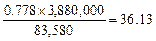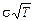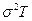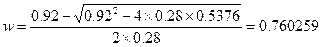Fundamentals of Futures and Options Markets, Seventh Edition

The following typos and other errors are in the first printing of the book and solutions manual. We have tried to correct them in subsequent printings and so they may not be present in the copy you have.

Errata in Book

Page 7: line 12, change “April” to “December” (This was kindly pointed out by Emilio Barone)

Page 13: Table 1.3: Change “Profit if April spot=1.9000” to “Profit if April spot=1.7000” and “Profit if April spot=1.8000” to “Profit if April spot equals 1.6000” (This was kindly pointed out by Bruce Valentine.)

Page 57: Example 3.4, second to last line: “Spot price on Nov. 10 + Gain on Futures” should be “Spot price on Nov. 10 − Gain on Futures” (This was kindly pointed out by Cheng-kun Kuo.)

Page 60: Final equation should bePage 77: line 6. Equation should be “a = 510 − 95×6= −60” (This was kindly pointed out by Emilio Barone)

Page 95: In Business Snapshot 4.3, 2-3 lines from end, change “million” to “billion”

Page 117: line 5. Change “Section 2.10” to “Section 2.11” (This was kindly pointed out by Emilio Barone)

Page 141: In Example 6.3, two lines from end change “0.9919” to “0.9913” (This was kindly pointed out by Emilio Barone)

Page 155: In Problem 6.25, line 1 change “December 2010” to “December 2011” (This was kindly pointed out by Emilio Barone)

Page 202: line 3, change “less than” to “greater than  (This was kindly pointed out by Edwin van Norden)

Page 202: The Mian and Sufi referenced article should be  The Consequences of Mortgage Credit Expansion: Evidence from the US Mortgage Default Crisis” Quarterly Journal of Economics, 124 (4), Nov 2009, 1449-96. (This was kindly pointed out by Emilio Barone)

Page 220: Line 1. Change “Section 2.9” to “Section 2.10” (This was kindly pointed out by Emilio Barone)

Page 238: Business Snapshot 10.1. Two lines from end. Change “equation (9.3)” to “equation (10.6)” (This was kindly pointed out by Emilio Barone)

Page 301: Example 13.4 should include the equation c=42N(0.7693)−38.048N(0.6278) before the equation for p.

Page 306: Example 13.6. d1 should be “0.2020” not “0.2017” and d2 should be “−0.0102”  not  “−0.0104”. The other numbers are OK. (This was kindly pointed out by Alexander Amati.)

Page 324: Problem 14.8. Change “Figure 14.2” to “Figure 14.1” (This was kindly pointed out by Emilio Barone)

Page 336: 3 lines above start of Section 15.5. change “forward rates” to “forward prices” (This was kindly pointed out by Emilio Barone)

Page 338: line 3: Change “(15.9) and (15.10)” to “(15.8) and (15.9)” (This was kindly pointed out by Emilio Barone)

Page 347: Two lines below equation (16.1). Change “equation (10.3)” to “equation (10.6)” (This was kindly pointed out by Emilio Barone)

Page 351: lines 5 and 6. Change “comparing equations (16.5) and (16.6) with equations (15.7) and (15.8)  to “comparing the equations in Section 16.6 with the equations in Section 15.6” (This was kindly pointed out by Emilio Barone and Chee Ng)

Page 352: Example 6.5: The answer is \$66.28, not \$44.19. The other numbers are correct. (This was kindly pointed out by Surendranath Jory)

Page 398: First line of Section 18.2. Delete “and 15.3” (This was kindly pointed out by Emilio Barone)

Page 412: In Problem 18.20 change “Example 18.1” to Example 18.3” and in Problem 18.21 change “Example 18.2” to “Example 18.4”

Page 441: 14 lines from end: change “standard deviation of the portfolio in equation (20.3)” to “variance of the portfolio in equation (20.3)” (This was kindly pointed out by Alex Saldarriaga.)

Page 469: last line of footnote. “0.2521” should be “0.2556” (This was kindly pointed out by Emilio Barone)

Page 483: Example 22.1, line 8. Change “equation (13.21)” to “equation (13.6)” (This was kindly pointed out by Emilio Barone)

Page 503: Change “billion” to “trillion” in the table in Business Snapshot 23.2

Page 589: url for Montreal exchange is www.m-x.ca

Errata in Solutions Manual

Page 7: Change “April” to “October” on lines 18, 21, 32, and 33 (This was kindly pointed out by Emilio Barone)

Page 23: Last line of Problem 4.15. Change “1964.67” to “2078.85” (This was kindly pointed out by Emilio Barone)

Page 29: Problem 5.13, last line. Delete the “United” (This was kindly pointed out by Emilio Barone)

Page 36: 2012 is a leap year and so calculations are  slightly off. The result is that “112.02” should be 112.03 at top of page 37. (This was kindly pointed out by Emilio Barone)

Page 80: Problem 13.8. Second argument of f should be 0.252 not 0.25 in two places. In Problem 13.9 second argument of f should be 0.352×0.5 in the first equation and 0.2472  in the second equation. (This was kindly pointed out by Emilio Barone)

Page 81: Problem 13.10. Second argument of f should benot(This was kindly pointed out by Emilio Barone)

Page 86: Problem 13.19. The implied volatility for the 3 month option with a strike price of 50 should be 32.12%, not 34.15%. (This was kindly pointed out by Surendranath Jory)

Page 87: Problem 13.21. Second argument of f should benot(This was kindly pointed out by Emilio Barone)

Page 133: Line 8. Change “equation (12.2)” to “equation (13.2)”. In the next three equations the second argument of f should be squared to become, 0.122×0.25, and 0.062, respectively.  (This was kindly pointed out by Emilio Barone)

Page 139: Second equation should be(This was kindly pointed out by Marco Salerno)

Page 147: Problem 21.16. On first line replace “Business Snapshot 21.2” with “Business Snapshot 21.1  (This was kindly pointed out by Emilio Barone)